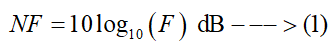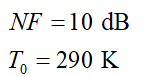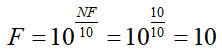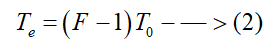# Noise Figure of an amplifier is given as 10 dB. If the ambient temperature is 290 K, then what will be the equivalent noise temperature?

Question
1 views

Noise Figure of an amplifier is given as 10 dB. If the ambient temperature is 290 K, then what will be the equivalent noise temperature?

check_circle

star
star
star
star
star
1 Rating
Step 1

The noise figure (NF) and noise factor (F) of an amplifier are related by the following equation:In this case, the values of the noise figure of the amplifier and the ambient temperature are given asStep 2

Using equation (1), the value of the noise factor of the amplifier will beStep 3

The equivalent noise temperature of the amplifier depends on the noise factor of the amplifier and it can be expressed as...

### Want to see the full answer?

See Solution

#### Want to see this answer and more?

Solutions are written by subject experts who are available 24/7. Questions are typically answered within 1 hour.*

See Solution
*Response times may vary by subject and question.
Tagged in

### Electrical Engineering